### Home > GC > Chapter 12 > Lesson 12.1.3 > Problem12-29

12-29.
1. Use the diagram of ⊙C below to answer the questions below it. Homework Help ✎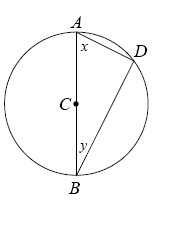1. If m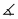x = 28°, what is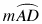?

2. If AD = 5 and BD =, what is the area of ⊙C?

3. If the radius of ⊙C is 8 and if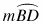= 100°, what is BD ?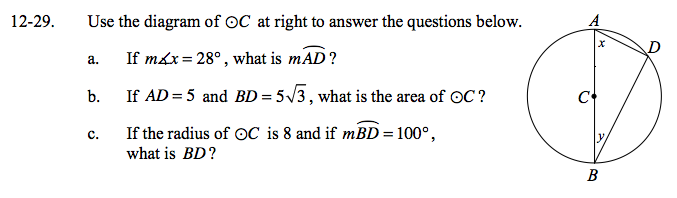D is a right angle. Why?

Use the Pythagorean Theorem to find the length of AB.
How can you use that length to find the area?

measure of arc AD = 124°

25π un2

Draw radius CD. Use the fact that ∠BCD = 100° and the Law of Sines to find BD.